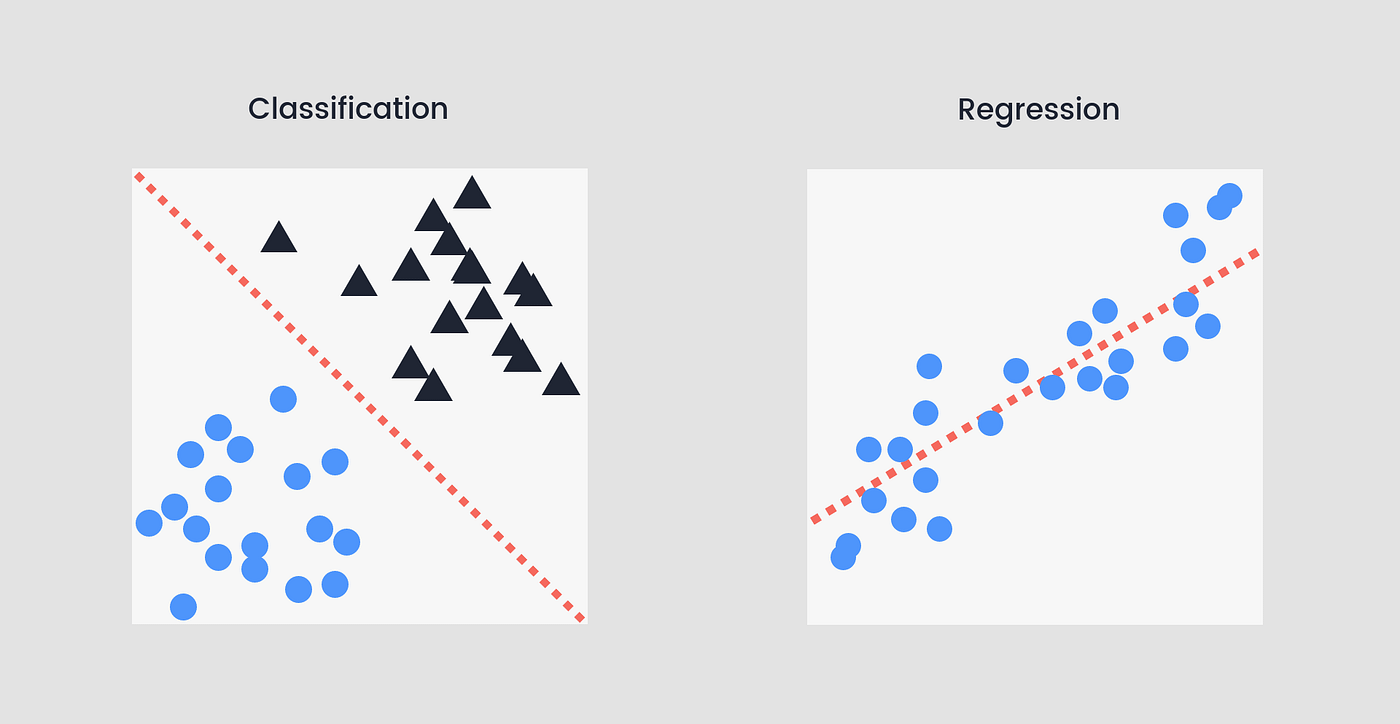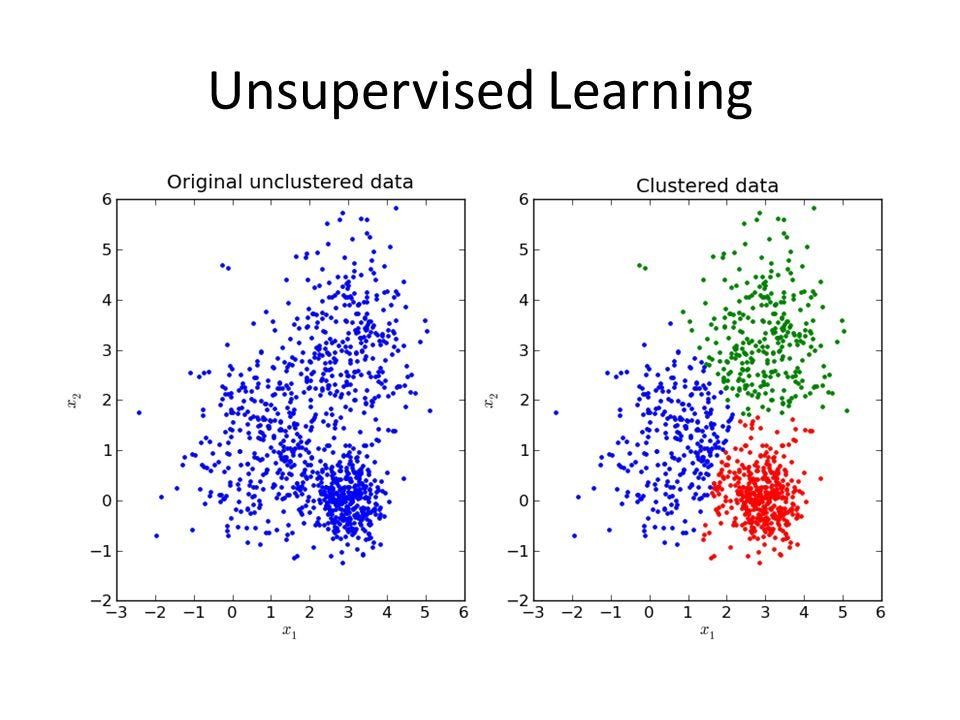In my previous article, we talked about DeepRacer and how we could all get into creating our own model. Here we will dive much deeper into how is DeepRacer learning from his actions, but also the different types of Machine Learning Algorithm available today.

# Machine Learning

Machine Learning (ML) is a broad term to talk about self-learning algorithms. It is used in multiple domains: engineering, retails, finance, commerce and more.

There are 3 families of learning. Supervised Learning is used to predict or classify labeled data. Unsupervised Learning is mainly used for clustering unlabelled data ( finding correlations ). And Reinforcement learning is used when an agent takes actions within an environment. For example an autonomous car within the city or a rescue-drone…

We are going to explain mostly what Reinforcement Learning (RL) is, but we first need to explain what are Supervised (SL) and Unsupervised Learning (UL) to understand best RL.

## Supervised Learning

In SL we teach the algorithm a model by giving a labeled dataset. It will create boundaries based on the dataset. It can produce a Classification ( classifying labeled data ) or a Regression ( predicting trends using previous data ). For example :

• Regression: predict a continuous numerical value. How much will that house sell for?
• Classification: assign a label. Is this a flat or a house?

##Now the maths about regression, How is the data used to approximate new outcomes ?. For those who a glimpse at an equation is harmful don’t worry, the text is here to guide you through.

First, we have an input ( multidimensional analysis is also possible ), let’s call it X, we want to predict the associated Y given an X. In a way, Y is a transformation of X.

This is why we make the hypothesis such as below. This should remind you of y = ax + b. This is the exact same thing as our equation except for the notation.### Our goal: Minimizing the error

Because we are making an estimation of a result, we can think about the error that is made. Of course, we all want to have the best approximation function. Therefore we want to minimize the cost function. Each x is corresponding to a y. The difference squared is the error that the hypothesis made.This iteration process is called Gradient Descent. This is an intuitive way of thinking about minimizing the cost. We want to change our parameters to end at the bottom of the bowl looking curve.On the graph, the X-axis (in red ) is θ0 and Y-axis (in green) is θ1. The Z-axis ( in blue ) is the cost function.

The equations are evil-looking, but this simply means that we tweak the θ corresponding value where this result is minimum. Why? Because we want to minimize the cost. So when we are at the bottom there is no better place to go. Therefore the algorithm stops and we have the best approximation function with parameters θ0 and θ1. Great!

### Linear regression

But, in real life, none have just one parameter. You have instead of one input X a bunch of inputs. Let’s say three, well, you go from two parameters to 4, for n inputs, you have n+1 parameters to tweak. This what it looks like, then with n inputs.Linear regression is only one example. On the same concepts, we can expand our parameters θ to deal with more complex behavior. For example, a multi-dimensional dataset following a curve can not be well-described as a line. Therefore we can add terms like in quadratics equations. But if the order of the function is too high, then it loses it’s the power to approximate new points.

We have it, a Supervised Learning algorithm able to predict with the best accuracy an outcome given a state. Some examples are to find across all fields, if there is an issue, there is a model that can help for sure.

## Unsupervised Learning

Unsupervised Learning is commonly referred to as Clustering ( finding patterns and groupings from unlabeled data ). This means that the algorithm is by itself extracting the useful information of an existing dataset. It can also help to reduce the dimensions of datasets, therefore used for data compression, filtering or parsing.

Some examples of unsupervised learning algorithms would be :

• An advertising platform segments the U.S. population into smaller groups with similar demographics and purchasing habits. => Advertisers can reach their target market with relevant ads.
• Airbnb groups its housing listings into neighborhoods. => Users can navigate listings more easily.

A data science team reduces the number of dimensions in a large data set to simplify the modeling and reduce file size.Example of a Clustered centroid-based ( K-means ) data

It exists many types of clustering algorithms:

• The K-Means is the simplest: taking the shortest data-set point and associating it with a centered group. But as you can see below this isn’t very accurate with every type of data.
• Therefore an OPTICS algorithm is used to avoid that.
• Still, an Elbow Method can be used to determine the optimal number of clusters ( with 2 below ) in any clustering algorithm.Example of Clustering via a centroid-based ( K-means ) algorithm versus a density-based ( OPTICS ) algorithm

As you can see, many different transformation and segmentation of data are possible. Indeed the strength of unsupervised learning algorithms is to see behind what humans can. They are capable of analyzing a multi-dimensional dataset, regrouping and reducing them to find a solution.

For more in-depth information about clustering analysis, check out this article.

## Reinforcement Learning

Before RL, we encountered situations where the data was already in a set file. All nice and comfy. But what if we want a more elaborated model, such that it is able to deal with the real world ( or a close simulation of it ).

In RL, there are 5 main parameters. The agent which interacts with his environment, he receives a reward and a new state based on the action the Agent did last time.We will focus on Proximal Policy Optimization (PPO), the algorithm used by AWS DeepRacer.

In the next diagram, two different neural networks are working in parallel. The Policy Network is the one who takes the action ( out of the Action space ) given a set of inputs ( likely an image, speed, steering, etc ). The Value Network predicts given a state the future reward. Here we train the algorithm to maximize the reward for a given environment by choosing the best action. This should ring a bell to you. The Value Network is predicting outcomes based on inputs, yes ! this is Supervised Learning.What is happening with PPO?  Well, the Value Network is trying to predict the reward given a state. When the action is taken gives a higher reward than expected, the probability of this action goes up in the Policy Network. Why? Because we want to maximize the reward. It is a sort of SL in the way that we are trying to get the best approximation of reward given a set of input and then taking the best action possible for maximum reward.

We start with a random weighted Policy Network, therefore the action taken is random. It produces a new state and a reward. It goes on for the set number of episodes. Next, after storing the experience we feed it to the algorithm. We then apply updates to the neural network. This is where we make the algorithm learn by tweaking the weights of the neural network. More infomation on that below.

What is a neural network? The Policy network and the value network are both neural nets, but what form do they take?

Each neuron is connected to all neurons from the previous and the next layer. This means that each connection can be tweaked to slightly change the result of the output layer. We want the algorithm to detect handwritten digits, therefore we want the certainty of the neural network to increase for the right answer and vice versa. All the weights of the vectors can be changed to get the desired output. That’s why, we define a neural network by the number of neurons in each layer, the function that each neuron applies to his input. And a matrice that associates each link from a neuron to another with a bias.

For example, a neural network can take this shape (mathematicly speaking ):The function used are squishing the outputs between 0 and 1 or this kind of operation. This speaks to me, because this is a straight operation, here this is a simple example but this stays the same for any size of neural network.

# Data produced and consumed in DeepRacer

With DeepRacer, data are generated by captors and transformed by AWS tools. Next is an example, extracted from the metrics in AWS S3:

```
"metrics":

"trial": 1
"completion_percentage": 40
"elapsed_time_in_milliseconds": 5958
"metric_time": 1564404356440
"start_time": 1564404350483

"trial": 2
"completion_percentage": 44
"elapsed_time_in_milliseconds": 6971
"metric_time": 1564404363947
"start_time": 1564404356976

"trial": 3
"completion_percentage": 42
"elapsed_time_in_milliseconds": 6896
"metric_time": 1564404371183
"start_time": 1564404364287

```

The Action Space is stored in S3 for each model, the same for the reward function. They are easy to locate, you can see them at the end of your file DeepRacer on S3.

Amazon SageMaker saves his data in S3, for each model, again we can find the action space, along with data in CSV and python script, this may be what is running the reinforcement learning.

Details of this model is not available from AWS. Maybe later…

Extract of the Episode CSV file :

```Episode #,Training Iter,In Heatup,ER #Transitions,ER #Episodes,Episode Length,Total steps,Epsilon,Shaped Training Reward,Training Reward,Update Target Network,Wall-Clock Time,Evaluation Reward,Shaped Evaluation Reward,Success Rate,Loss/Mean,Loss/Stdev,Loss/Max,Loss/Min,Learning Rate/Mean,Learning Rate/Stdev,Learning Rate/Max,Learning Rate/Min,Grads (unclipped)/Mean,Grads (unclipped)/Stdev,Grads (unclipped)/Max,Grads (unclipped)/Min,Discounted Return/Mean,Discounted Return/Stdev,Discounted Return/Max,Discounted Return/Min,Entropy/Mean,Entropy/Stdev,Entropy/Max,Entropy/Min,Advantages/Mean,Advantages/Stdev,Advantages/Max,Advantages/Min,Values/Mean,Values/Stdev,Values/Max,Values/Min,Value Loss/Mean,Value Loss/Stdev,Value Loss/Max,Value Loss/Min,Policy Loss/Mean,Policy Loss/Stdev,Policy Loss/Max,Policy Loss/Min,Value Targets/Mean,Value Targets/Stdev,Value Targets/Max,Value Targets/Min,KL Divergence/Mean,KL Divergence/Stdev,KL Divergence/Max,KL Divergence/Min,Likelihood Ratio/Mean,Likelihood Ratio/Stdev,Likelihood Ratio/Max,Likelihood Ratio/Min,Clipped Likelihood Ratio/Mean,Clipped Likelihood Ratio/Stdev,Clipped Likelihood Ratio/Max,Clipped Likelihood Ratio/Min

1,0.0,0.0,18.0,1.0,18.0,18.0,0.0,16.991097745197745,16.991097745197745,0.0,0.0,4.64327892008701,6.443740360739934,16.92444316780398,-2.79730089002997

2,0.0,0.0,52.0,2.0,34.0,52.0,0.0,14.323455671263769,14.323455671263769,0.0,2.955521821975708,3.5338439316704533,4.035311362590394,14.217522500087062,-3.79450358913994

3,0.0,0.0,75.0,3.0,23.0,75.0,0.0,24.361157157474775,24.361157157474775,0.0,5.139806509017944,7.008071248702334,10.37061366777746,24.224195714469072,-5.48621827646525;

4,0.0,0.0,91.0,4.0,16.0,91.0,0.0,2.669900132699191,2.669900132699191,0.0,6.85621190071106,-1.467218417183811,1.9198072528942156,2.6960716990732068,-3.79440368913994

5,0.0,0.0,109.0,5.0,18.0,109.0,0.0,22.114211844486938,22.114211844486938,0.0,8.612297534942627,4.939842572757495,7.547080051305869,22.0473420201975,-3.79410398903994

6,0.0,0.0,129.0,6.0,20.0,129.0,0.0,14.95392513103146,14.95392513103146,0.0,10.633955717086792,1.384037132202164,5.349929199567146,14.941185573961375,-5.58581887606535

7,0.0,0.0,148.0,7.0,19.0,148.0,0.0,16.924193383890657,16.924193383890657,0.0,12.40947961807251,2.175092013249806,6.347386792950399,16.899766402040935,-3.9940039890399404

#Quick note: for some readability, exentrics writting has been removed but the data remains extremely similar from the original
```

So, SageMaker uses a Redis file applying the python code from the adjacent .py file, so the CSV file is a sum-up of all the data extracted when learning. It must be edited at each step of the backpropagation algorithm ( learning algorithm ). Then a new model is created and stored in a parallel file using the previous model as a “template”. Then the model is sent to RoboMaker, which sends its data to Redis and the cycle goes on and on until max iteration.

# Conclusion

This concludes this quick explanation of the inner workings of AWS DeepRacer, but more especially Machine Learning in general. Dealing with Supervised, Unsupervised and Reinforcement Learning.

#### Sources

Machine Learning with DeepRacer